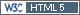## Two PK metrics: Inflation of the Type I Error [Two-Stage / GS Designs]

Dear all,

related to this thread about dropouts. To run the R-code you need package `Power2Stage` 0.4.6+.

Let’s assume a CV of 25% for Cmax and 15% for AUC, Potvin ‘Method B’ (αadj 0.0294). We want to play it safe and plan the first stage like a fixed sample design (T/R 0.95, 80% power). Hence, we start with 28 subjects. In the interim the CVs are higher than expected; for Cmax a CV of 30% and for AUC 20%. Say Cmax is not BE (94.12% CI) and power <80%. Hence, we should initiate the second stage. Re-estimated sample size:

```library(Power2Stage) print(sampleN2.TOST(CV=0.30, n1=28), row.names=FALSE) # Cmax # Design  alpha  CV theta0 theta1 theta2 n1 Sample size Achieved power Target power #    2x2 0.0294 0.3   0.95    0.8   1.25 28          20      0.8177478          0.8```

What does that mean? We would initiate the second stage with 20 subjects for Cmax but possible shouldn’t for AUC:

```print(sampleN2.TOST(CV=0.20, n1=28), row.names=FALSE) # AUC # Design  alpha  CV theta0 theta1 theta2 n1 Sample size Achieved power Target power #    2x2 0.0294 0.2   0.95    0.8   1.25 28           0      0.8922371          0.8```

Since the Type I Error strongly depends on the sample size, the study would be overrun for AUC and an inflated TIE is quite possible. If have no R-code yet to estimate how much… Suggestions are welcome.
I think that in the past everybody (including myself) looked only at the PK metric with the highest variability and ignored the other one. Likely not a good idea.

Which options do we have for the PK metric with the lower variability?
1. Assess BE with a lower sample size. In the example above ignore the second stage entirely. If the CV would be 25% instead of 20%, assess only the first six subjects of the 20 in the second stage (i.e., in the pooled analysis 28+6=34 instead of 48).
2. Use the data of all subjects and adjust α more (i.e., a wider CI). How?
3. Or?
#1 would preserve the TIE but would regulators accept it (not using all available data)? What about #2, when the GL tells us that the α has to be pre-specified in the protocol?

Of course, this issue is not limited to TSDs but applies to GSDs with (blinded/unblinded) sample size re-estimation as well.

Cheers,
Helmut SchützThe quality of responses received is directly proportional to the quality of the question asked. ☼
Science QuotesIng. Helmut Schütz